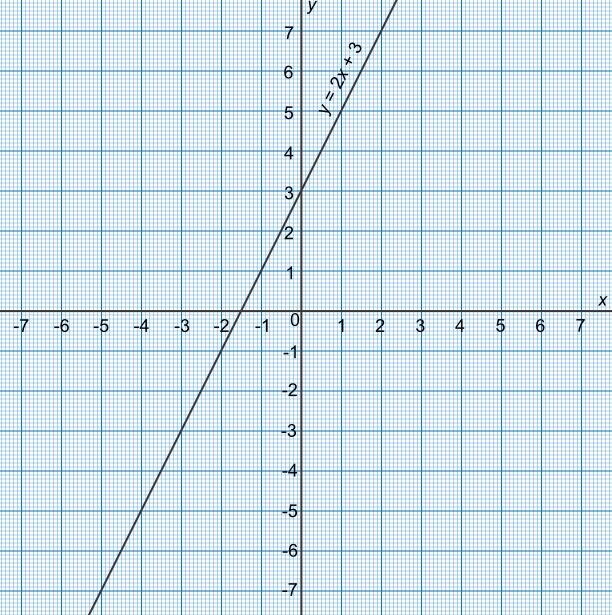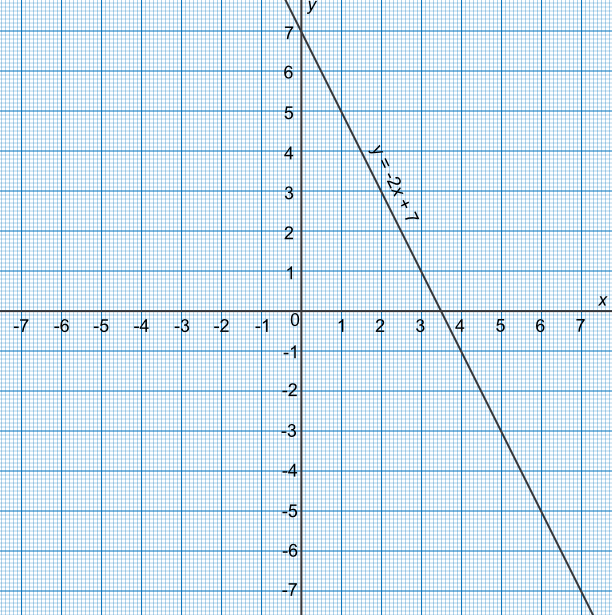Using Graphs to Solve Equations

## Using Graphs to Solve Equations

Graphs can be used to estimate solutions to equations.

The solution to the equation is given where the graph crosses the x-axis.

For some types of graphs, such as quadratics, there may be more than one solution if the line crosses the x-axis more than once. There may also be no solutions if the the line does not cross the x-axis at all.

When a solution is found, substitute back into the equation to check the answer.

In order to plot the graph correctly, you may have to re-arrange the equation as one side of the equation must equal zero.

## Example 1

The graph shows the function 2x + 3. What is the solution to 2x + 3 = 0?The line crosses the x-axis at - 1.5

Check the answer: 2(-1.5) + 3 = 0✔

Answer: x = -1.5

## Example 2

By drawing a graph, obtain the solution for -2x + 10 = 3.

It is necessary for one side of the equation to equal zero

Subtract 3 from both sides of the equation to get -2x + 7 = 0

Plot the graph using three points

The line crosses the x-axis at x = 3.5

Check the answer: -2(2.5) + 10 = 3✔Answer: x = 3.5